Next: 2.3.2 Time Discretization Up: 2.3 Constitutive Relation Formulation Previous: 2.3 Constitutive Relation Formulation

## 2.3.1 Thermal Simulation

The standard way of treating temperature effects in semiconductor devices and circuits is based on the assumption of a constant device temperature which can be obtained by a priori assumptions on the dissipated power or by measurements. However, in general this a priori assumed dissipated power is not in accordance with the resulting dissipated power. Furthermore, devices may be thermally coupled resulting in completely different temperatures than would be expected from individual self-heating effects alone. This is of special importance as many circuit layouts rely on this effect, e.g., current mirrors and differential pairs . Therefore, the temperature must not be considered a constant parameter, but must be introduced as an additional solution variable.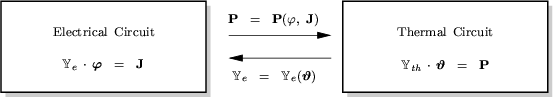Thermal coupling can be modeled by a thermal circuit [25,45]. The topological equations describing a thermal circuit are similar in form to Kirchhoff's equations and the branch relations map to familiar electrical branch relations. The electrical compact models have been extended to provide the device temperature as an external node. For distributed devices MINIMOS-NT solves the lattice heat flow equation to account for self-heating effects. This is of course far more accurate than assuming a spatially constant temperature in the device and estimating the dissipated power by Joule-heat terms alone as is done for the compact models. To provide a connection to an external thermal circuit arbitrary thermal contacts can be defined.

The thermal heat flow P between two points with temperatures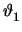and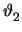is given by 

 P = Gth . (-) (2.25)

with Gth being the thermal conductance between these two points. In addition, the heat W stored in a device is proportional to the temperature difference

 W = Cth . (-) (2.26)

with Cth being the thermal capacitance. As the heat flow H is the derivative of W with respect to time one gets

 H =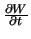= Cth .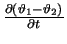(2.27)

Equation (2.25) is equivalent to Ohm's law with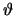replacing the node voltage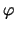and H replacing the branch current I between these two nodes, and (2.27) is equivalent to an electrical capacitor. Hence the same methods as derived above can be used to model thermal interaction between circuit elements. The solution vector x is augmented by the node temperature vectorand the branch heat flow vector P.Next: 2.3.2 Time Discretization Up: 2.3 Constitutive Relation Formulation Previous: 2.3 Constitutive Relation Formulation
Tibor Grasser
1999-05-31